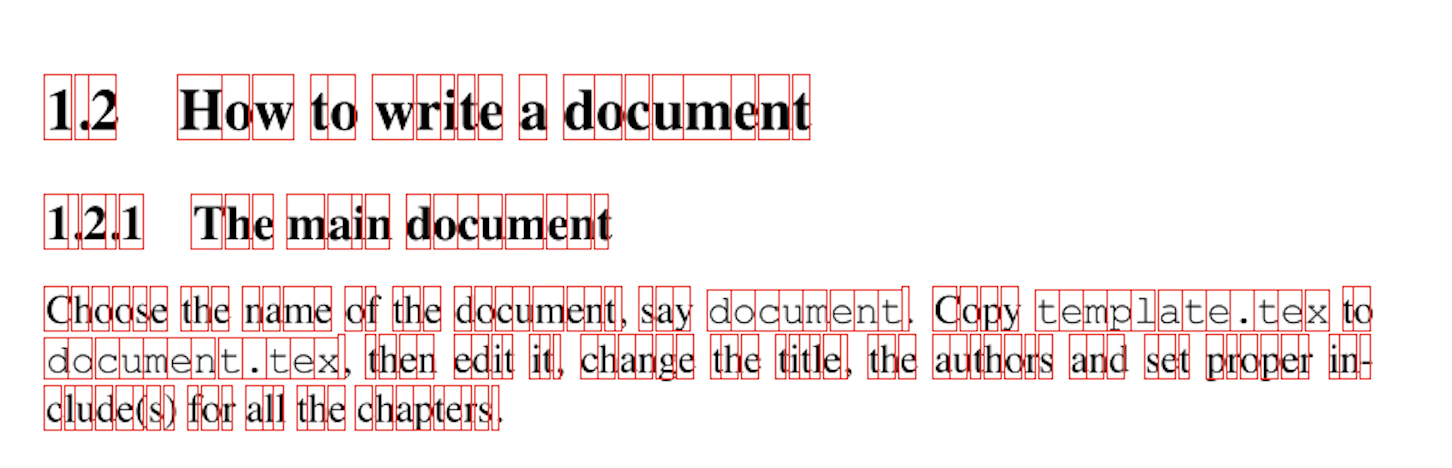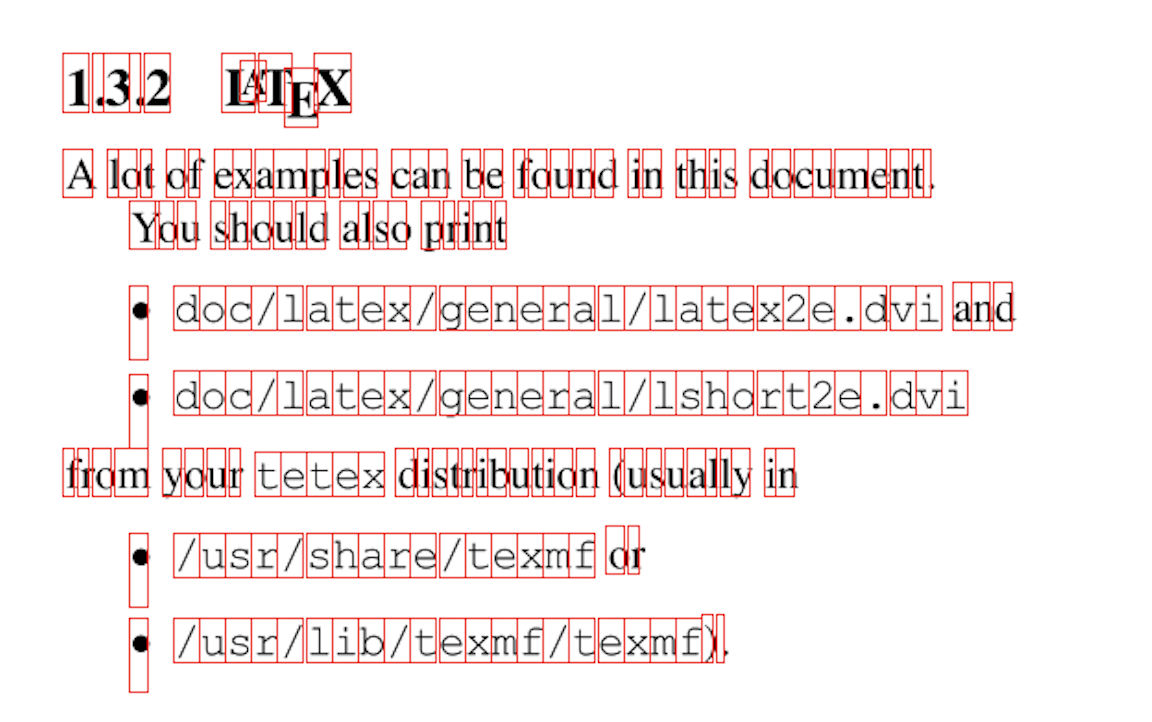# Extracting Text and Bounding Boxes from a PDF

This is part one of a series of blog posts where I explain how BankStatementConverter works. In this post I’m going to explain the code that figures out the bounding boxes and other attributes of characters on a page. Lots of this code was lifted from DrawPrintTextLocations and PDFTextStripper.

First thing we do is load the PDF file using PDFBox and then we process the document page by page. The PDFs are processed page by page because we don’t run out of memory, most documents are less than ten pages long, but there are documents out there that are over 10,000 pages long, if we tried to load all the data from a large document into memory we would quickly run out and crash our app. We start the parsePage method by extracting all the characters on the page so that we end up with a list of CharAndBound objects.

``````data class CharAndBound(
val char: Char,
val bound: Rectangle,
val color: Int,
val fontCode: Int,
val rotation: Int
) : IRectangle by bound
``````

### FastCharacterParser

The stripPage method of the FastCharacterParser class is responsible for returning a list of CharAndBound objects. It extends PDFBox’s PDFStreamEngine class and listens to the following events:

``````private fun setOperators() {
}
``````

As PDFStreamEngine comes across a glyph it calls the showGlyph method in FastCharacterParser, this is where we calculate all the properties of the CharAndBound object.

``````override fun showGlyph(
textMatrix: Matrix,
font: PDFont,
code: Int,
displacement: Vector
)
``````

We can use the textMatrix parameter to determine the rotation and the position of the glyph on the page. We also want the width and the height of the glyph, we use the bounding box of the font to determine that. Some fonts do not have bounding box information, when that happens we fallback to a hardcoded bounding box and hope for the best.

``````val bbox = getBoundingBoxWithHackyFallback(font)

// advance width, bbox height (glyph space)
val rectangle = Rectangle2D.Float(0f, bbox.lowerLeftY, xAdvance, bbox.height)
``````

At this point we have a Rectangle2D, but it is in ‘glyph space’ instead of ‘document space’, one obvious problem you can see above is the x coordinate is hardcoded to 0f. The next step is to transform this rectangle from glyph space to document space.

``````val affineTransform = textMatrix.createAffineTransform()

// Transform the rectangle
val buffer = floatArrayOf(rectangle.x, rectangle.y, rectangle.x + rectangle.width, rectangle.y + rectangle.height)
affineTransform.transform(buffer, 0, buffer, 0, 2)
flipAT.transform(buffer, 0, buffer, 0, 2)
rotateAT.transform(buffer, 0, buffer, 0, 2)

// Calculate the bounds of the transformed rectangle
val minX = min(buffer, buffer)
val maxX = max(buffer, buffer)
val minY = min(buffer, buffer)
val maxY = max(buffer, buffer)
val bound = Rectangle(minX, minY, maxX - minX, maxY - minY)
``````

The AffineTransformations above are configured based on the rotation of the page and the height of the page. PDFs define their origin as the bottom left of the page, I prefer a top left origin and flipAT performs that translation. Similarly rotateAT is responsible for adding page rotation to the bounding box of the character. Most documents have a rotation of 0, but often landscape documents have a rotation of 90 or 270. Now we have the bounding box of the glyph on the page. Next we need to figure out what character(s) this font code represents.

``````var unicode = font.toUnicode(code, glyphList)

if (unicode == null) {
if (font is PDSimpleFont) {
val c = code.toChar()
unicode = String(charArrayOf(c))
} else {
return
}
}

unicode = normalizeWord(unicode)
``````

First we try get the characters for the fontCode from the font. If that fails, and the font is a SimpleFont we assume the font’s code is unicode and just convert it to a character. The normalizeWord method is responsible for breaking up composed characters their base parts. The character ‘ﬀ’ is encoded in UTF16 as FB00, normalizeWord breaks it up into two ‘f’ characters and returns the string “ff”.

``````if (unicode.length == 1) {
return
}

val startX = bound.left()
val width = bound.width / unicode.length

for (index in unicode.indices) {
val x = startX + (index * width)
characters.add(CharAndBound(unicode[index], bound.copy(x = x, width = width), rgb, fontCode, rotation))
}
``````

Usually the String formed from the characterCode is of length one. If that’s the case we can generate a CharAndBound and add it to the list of characters. However if we come across a composed character like ‘ﬀ’, unicode will be “ff” and have a length of two. The code above shares the bounding box across the characters in the string. For example assume ‘ﬀ’ had a bounding box of [0,0,120,30], after splitting the first ‘f’ would have a bounding box of [0,0,60,30] and the second ‘f’ would have a bounding box of [60,0,60,30]. This code doesn’t work for vertical text, although vertical text seems safe to ignore for my use case.

Now let’s see it in action, I found this PDF online, extracted the bounding boxes and then rendered them onto each page. They look accurate enough for our purposes.### Next Time

In part two, we’ll talk about how we use the CharAndBound list to determine the headers of a transaction table.